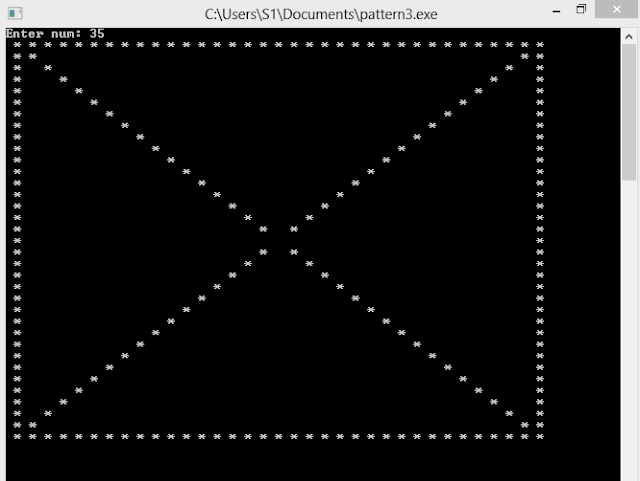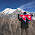### Print pattern in c - explore new perspectives in pattern printing## You will be able to :

• >> Design any kind of pattern
• >>Understand element of pattern designing
• >>Understand patterns
• >>Understand loop in c
• >>Understand Sequence analysis

## What is Pattern?

A pattern is set of  same or different kind of things that represent definite geometrical shapes or size.

Geometrical shape can be
• Triangle
• Square
• Pramid
If you look above picture than difinetly you will find repetition of shapes or color in definite manner .

It's example of pattern.
For Creating sucessfull Pattern , You must know

• Loop
• Element of pattern
• Sequence analysis of pattern

## Loop

loop are the control statement .

They are used when programmer needs to execute certain part of program for definite repetation

Different kinds of loop are
• For loop
• While loop
• Do while loop
Among these for loop is used when the number of repetition is known.

Although while and do-while loop can be used while number of loop is known but it is a bit complex

As above explained Pattern contain certain repitation and that is known , so we will use for loop for building patterns

### Syntax- for loop

``` for ( init; condition; increment )

{

statement(s);

}```
Here is the flow of control in a 'for' loop −

•  The init step is executed first, and only once. This step allows you to declare and initialize any loop control variables. You are not required to put a statement here, as long as a semicolon appears.
• Next, the condition is evaluated. If it is true, the body of the loop is executed. If it is false, the body of the loop does not execute and the flow of control jumps to the next statement just after the 'for' loop.
• After the body of the 'for' loop executes, the flow of control jumps back up to the increment statement. This statement allows you to update any loop control variables. This statement can be left blank, as long as a semicolon appears after the condition.
• The condition is now evaluated again. If it is true, the loop executes and the process repeats itself (body of loop, then increment step, and then again condition). After the condition becomes false, the 'for' loop terminates.

## Element of Pattern

There are number of element that should be well understood before building a pattern.

### Number of row and column

Number of column represent number of repetition of first loop first loop with five-time repetition.
Code of above program contain

```#include
int main()
{
int i, j;
for(i=5;i>=1;i--)
{    //other code
}
return 0;
}
```

Similarly each row are handled in each repetation of first loop.

### Character used

Number of different types of character denote the number of loop inside first loop.i.e nested loop.
In above pattern we have to print only '*' , so it will have only one nested lopp.
But in above pattern it contain two nested loop with other for easy.

## Sequence analysis

It is very important and easy to analysis pattern

This is the combination of * and blank space.  we have to  identify that in which position we need to print *and don't worry about the blank spaces.

we can analysis like this

[ "-" represent a blank space][ and n=9]

## Step By Step Solution

1.this program is a shape of matrix. so we need to use two for loop to solve this problem.
``` for(i=1;i<=n;i++)
{
for(j=1;j<=n;j++)
{
if(condition)
printf(" *");
else
printf("  ");//blank space

}
printf("\n");
}```
2. ith 1st and n(9)th index jth 1st and n(9)th index you see. each position we need to print '*' . in side loop condition we can write it as..
```      if(i==1 || i==n || j==1 || j==n)
printf(" *");
else
printf("  ");```
then output come like box.
3. now we need to draw like:
here we can see it's printing * where-where ith index and jth index are equals except middle mean [(n/2)+1=5] so we can write code for this:
```        if(i==j && j!=(n/2)+1)
printf(" *");
else
printf("  ");
```
4. Now last pattern, reverse of step(iii) pattern.. ex:

5. so here we can analyse it's decreasing order of j index 9,8,7,6,4,3,2,1 except middle means [n/2+1=5] For this we can write the condition:-

```       if(j==(n+1)-i && j!=(n/2)+1)
printf(" *");
else
printf("  "); ```

In above example we declared the some variation of pattern based on condition... Even we combined 3 and 4 condition then we will get output like:

condition:
```       if(i==j && j!=(n/2)+1 || j==(n+1)-i && j!=(n/2)+1)
printf(" *");
else
printf("  ");
```

And finally Even we combined this 5 with 2 then we will get output like: Final Example:

so final code is:

```#include
#include

int main()
{
int n , i ,j;

printf("Enter number: ");
scanf("%d",&n);
//first loop
for( i=1;i<=n;i++)
{
for(int j=1;j<=n;j++)
{

if(i==1 || j==1 || i==n|| j==n || i==j && j!=(n/2)+1 || j==((n+1)-i) && j!=n/2+1)
{
printf(" *");
}
else
printf("  ");
}
printf("\n");
//next line
}
//pattern end
getch();
}```

## SUMMERY

1. A pattern of numbers, star or characters is a way of arranging these in some logical manner or they may form a sequence
2. Some of these patterns are triangles which have special importance in mathematics. Some patterns are symmetrical while other are not.
3. Creating a pattern involves how to use nested loops properly, some pattern may involve alphabets or other special characters. Key aspect is knowing how the characters in pattern changes.
4.  pattern is set of  same or different kind of things that represent deifinite geometrical shhapes or size.
5. the important element of pattern is it'e order or sequence.
6. you must be able to recognize order or sequence of pattern inorder to build it.
Below video can be helpful for understanding Pattern

## DO NOT MISS OTHER C PROGRAMMING TUTORIAL

* indicates required

You can Browse related article below for more information and program code related to pattern

Does above is helpful , Post you views in comment

1.Print star pattern in c - explore new perspectives in pattern designing is a very nice article thanks . please keep posting such great article

1.Thanks for your response in Print star pattern in c - explore new perspectives in pattern designing

2.12345
1234
123
12
1

1.int i, j;
for(i=5;i>=1;i--)
{
for(j=1;j<=i;j++)
{
printf("%d",j);
}
printf("\n");
}

3.how to print below pattern help

12344321
123**321
12****21
1******1

1.int i,j,k;
for(i=4;i>=1;i--)
{
for(j=1;j<=4;j++)
{
if(j<=i)
printf("%d",j);
else
printf(" ");
}
for(j=4;j>=1;j--)
{
if(j<=i)
printf("%d",j);
else
printf(" ");
}
printf("\n");
}